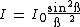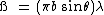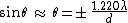diffraction

(redirected from diffracts)
Also found in: Dictionary, Thesaurus, Medical.
Related to diffracts: refracted

diffraction,

bending of waves around the edge of an obstacle. When light strikes an opaque body, for instance, a shadow forms on the side of the body that is shielded from the light source. Ordinarily light travels in straight lines through a uniform, transparent medium, but those light waves that just pass the edges of the opaque body are bent, or deflected. This diffraction produces a fuzzy border region between the shadow area and the lighted area. Upon close examination it can be seen that this border region is actually a series of alternate dark and light lines extending both slightly into the shadow area and slightly into the lighted area. If the observer looks for these patterns, he will find that they are not always sharp. However a sharp pattern can be produced if a single, distant light source, or a point light source, is used to cast a shadow behind an opaque body. Diffraction also occurs when light waves interact with a device called a diffraction grating. A diffraction grating may be either a transmission grating (a plate pierced with small, parallel, evenly spaced slits through which light passes) or a reflection grating (a plate of metal or glass that reflects light from polished strips between parallel lines ruled on its surface). In the case of a reflection grating, the smooth surfaces between the lines act as narrow slits. The number of these slits or lines is often 12,000 or more to the centimeter (30,000 to the inch). The ruling is generally done with a fine diamond point. Since the light diffracted is also dispersed (see spectrumspectrum,
arrangement or display of light or other form of radiation separated according to wavelength, frequency, energy, or some other property. Beams of charged particles can be separated into a spectrum according to mass in a mass spectrometer (see mass spectrograph).
), these gratings are utilized in diffraction spectroscopesspectroscope,
optical instrument for producing spectral lines and measuring their wavelengths and intensities, used in spectral analysis (see spectrum). When a material is heated to incandescence it emits light that is characteristic of the atomic makeup of the material.
for producing and analyzing spectra and for measuring directly the wavelengths of lines appearing in certain spectra. The diffraction of X rays by crystals is used to examine the atomic and molecular structure of these crystals. Beams of particles can also exhibit diffraction since, according to the quantum theoryquantum theory,
modern physical theory concerned with the emission and absorption of energy by matter and with the motion of material particles; the quantum theory and the theory of relativity together form the theoretical basis of modern physics.
, a moving particle also has certain wavelike properties. Both electron diffraction and neutron diffraction have been important in modern physics research. Sound waves and water waves also undergo diffraction.

Diffraction

The bending of light, or other waves, into the region of the geometrical shadow of an obstacle. More exactly, diffraction refers to any redistribution in space of the intensity of waves that results from the presence of an object that causes variations of either the amplitude or phase of the waves. Most diffraction gratings cause a periodic modulation of the phase across the wavefront rather than a modulation of the amplitude. Although diffraction is an effect exhibited by all types of wave motion, this article will deal only with electromagnetic waves, especially those of visible light. For discussion of the phenomenon as encountered in other types of waves See Electron diffraction, Neutron diffraction, Sound

Diffraction is a phenomenon of all electromagnetic radiation, including radio waves; microwaves; infrared, visible, and ultraviolet light; and x-rays. The effects for light are important in connection with the resolving power of optical instruments. See X-ray diffraction

There are two main classes of diffraction, which are known as Fraunhofer diffraction and Fresnel diffraction. The former concerns beams of parallel light, and is distinguished by the simplicity of the mathematical treatment required and also by its practical importance. The latter class includes the effects in divergent light, and is the simplest to observe experimentally.

To illustrate the difference between the methods of observation of the two types of diffraction, Fig. 1 shows the experimental arrangements required to observe them for a circular hole in a screen S. The light originates at a very small source O, which can conveniently be a pinhole illuminated by sunlight. In Fraunhofer diffraction, the source lies at the principal focus of a lens L1 which renders the light parallel as it falls on the aperture. A second lens L2 focuses parallel diffracted beams on the observing screen F, situated in the principal focal plane of L2. In Fresnel diffraction, no lenses intervene. The diffraction effects occur chiefly near the borders of the geometrical shadow, indicated by the broken lines. An alternative way of distinguishing the two classes, therefore, is to say that Fraunhofer diffraction concerns the effects near the focal point of a lens or mirror, while Fresnel diffraction concerns those effects near the edges of shadows. Photographs of diffraction patterns are shown in Fig. 2.

Fraunhofer diffraction

This class of diffraction is characterized by a linear variation of the phases of the Huygens secondary waves with distance across the wavefront, as they arrive at a given point on the observing screen. At the instant that the incident plane wave occupies the plane of the diffracting screen, it may be regarded as sending out, from each element of its surface, a multitude of secondary waves, the joint effect of which is to be evaluated in the focal plane of the lens L2. The analysis of these secondary waves involves taking account of both their amplitudes and their phases. The simplest way to do this is to use a graphical method, the method of the so-called vibration curve, which can readily be extended to cases of Fresnel diffraction. See Huygens' principle

The vibration curve results from the addition of a large (really infinite) number of infinitesimal vectors, each representing the contribution of the Huygens secondary waves from an element of surface of the wavefront. If these elements are assumed to be of equal area, the magnitudes of the amplitudes to be added will all be equal. They will, however, generally differ in phase, so that if the elements were small but finite each would be drawn at a small angle with the preceding one, as shown in Fig. 3a. The resultant of all elements would be the vector A. When the individual vectors represent the contributions from infinitesimal surface elements (as they must for the Huygens wavelets), the diagram becomes a smooth curve, the vibration curve, shown in Fig. 3b. The intensity on the screen is then proportional to the square of this resultant amplitude. In this way, the distribution of the intensity of light in any Fraunhofer diffraction pattern may be determined.

The intensity distribution for Fraunhofer diffraction by a slit as a function of the angle Θ from the center may be simply calculated by the method of the vibration curve. The intensity at any angle is given by Eq. (1), where I0 is the intensity at the center of the pattern, and ß is given by Eq. (2), where b is the

(1)(2)width of the slit and λ is the wavelength. The central maximum is twice as wide as the subsidiary ones, and is about 21 times as intense as the strongest of these. A photograph of this pattern is shown in Fig. 2a.

Fraunhofer diffraction by a circular aperture determines the resolving power of instruments such as telescopes, cameras, and microscopes, in which the width of the light beam is usually limited by the rim of one of the lenses. The method of the vibration curve may be extended to find the angular width of the central diffraction maximum for this case. An exact construction of the curve or, better, a mathematical calculation shows that the extreme phase differences required are ± 1.220π, yielding

(3)Eq. (3) for the angle Θ at the first zero of intensity. Here d is the diameter of the circular aperture. This pattern has circular symmetry and consists of a diffuse central disk, called the Airy disk, surrounded by faint rings. The angular radius of the disk, given by Eq. (3), may be extremely small for an actual optical instrument, but it sets the ultimate limit to the sharpness of the image, that is, to the resolving power. See Resolving power (optics)

Fresnel diffraction

The diffraction effects obtained when the source of light or the observing screen are at a finite distance from the diffracting aperture or obstacle come under the classification of Fresnel diffraction. This type of diffraction requires for its observation only a point source, a diffracting screen of some sort, and an observing screen. The latter is often advantageously replaced by a magnifier or a low-power microscope. The observed diffraction patterns generally differ according to the radius of curvature of the wave and the distance of the point of observation behind the screen. If the diffracting screen has circular symmetry, such as that of an opaque disk or a round hole, a point source of light must be used. If it has straight, parallel edges, it is desirable from the standpoint of brightness to use an illuminated slit parallel to these edges. In the latter case, it is possible to regard the wave emanating from the slit as a cylindrical one. For the purpose of deriving the vibration curve, the appropriate way of dividing the wavefront into infinitesimal elements is to use annular rings in the first case, and strips parallel to the axis of the cylinder in the second case.

The zone plate is a special screen designed to block off the light from every other half-period zone, and represents an interesting application of Fresnel diffraction. The Fresnel half-period zones are drawn, with radii proportional to the square roots of whole numbers, and alternate ones are blackened. The drawing is then photographed on a reduced scale. When light from a point source is sent through the negative, an intense point image is produced, much like that formed by a lens.

diffraction

(di-frak -shŏn) The bending of light or other electromagnetic radiation at the edges of obstacles, with the resulting formation of light and dark bands or rings (diffraction patterns) at the edges of the shadow. An example of a diffraction pattern is the Airy disk observed in telescope images of distant celestial objects. A small aperture or slit produces similar annular or banded patterns. Diffraction results from the wave nature of electromagnetic radiation. See also interference.

diffraction

[di′frak·shən]
(physics)
Any redistribution in space of the intensity of waves that results from the presence of an object causing variations of either the amplitude or phase of the waves; found in all types of wave phenomena.

diffraction

1. Physics a deviation in the direction of a wave at the edge of an obstacle in its path
2. any phenomenon caused by diffraction and interference of light, such as the formation of light and dark fringes by the passage of light through a small aperture
3. deflection of sound waves caused by an obstacle or by nonhomogeneity of a medium

diffraction

The bending of electromagnetic waves as they pass around corners or through holes smaller than the wavelengths of the waves themselves. See diffraction grating and refraction.
References in periodicals archive ?
In the absence of glucose, the sensor diffracts 640 run red light (Fig.
If another token arrives, the processor diffracts the two tokens, sending one in a message to the left balancer and the other in a message to the right.
Passing through the windows, the fields can then be diffracted through 90 |degrees~ by the window frame, and thereby propagate vertically along the face of the building, At windows on other floors, the field diffracts back into the building, finally reaching the receiver.
The method of claim 1, wherein the array of elements comprises an ordered array of particles, and wherein the first cured portion diffracts radiation at a first wavelength and the other cured portion diffracts radiation at another wavelength.
By definition all quasi-periodic objects diffract discretely, even though they are not periodic.
Because light bends, or diffracts, around the edges of objects, ordinary optical microscopes can discern features no closer than a half-wavelength apart, a distance of 200 nanometers (nm) or so.
In a combination of an article having a hologram or diffraction grating device attached to a surface thereof, said device being characterized by a surface relief pattern that reflects and diffracts incident light from portions backed by a reflective layer to form an image or other light pattern recorded therein, an improvement wherein said reflective layer includes at least a portion thereof only partially covering the surface relief pattern in a shape that conveys visual information separate from that of said image or other light pattern.
The team can also deposit a gold pattern, which diffracts light differently as the gel shrinks and swells with changing temperatures.
They derive from interference patterns created when light diffracts as it passes through a two-dimensional grid.

Site: Follow: Share:
Open / Close